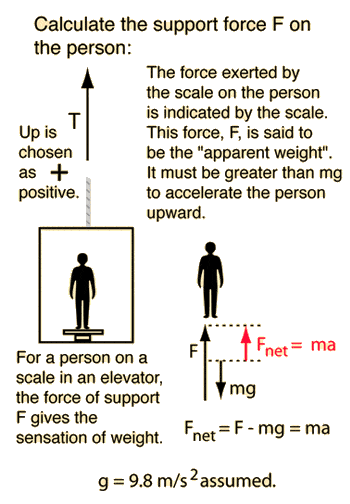# Acceleration in an elevator

• zellster87

## Homework Statement

You've always wondered about the acceleration of the elevators in the 101-story-tall Empire State Building. One day, while visiting New York, you take your bathroom scale into the elevator and stand on it. The scale reads 150 lbs as the door closes. The reading varies between 120 lbs and 170 lbs as the elevator travels 101 floors.

a.)What is the maximium acceleration upward?
b.)What is the maximium magnitude of the acceleration downward?

f=mg

## The Attempt at a Solution

First of all, i don't understand how the reading on the scale can vary under their weight if the elevator is going up. It seems to me that unless the acceleration is negative, their weight should be positive throughout going up. Where is my logic wrong?

For a mass m= kg, the elevator must support its weight = mg =Newtons to hold it up at rest. If the acceleration is a=m/s² then a net force=Newtons is required to accelerate the mass. This requires a support force of F=Newtons. Note that the support force is equal to the weight only if the acceleration is zero, and that if the acceleration is negative (downward), the support force is less than the weight. If you enter a downward acceleration greater than 9.8 m/s² you will get a negative support force, showing that you must force it downward to get an acceleration greater than that of free fall.#### Attachments

Ah ok, I got it. Thanks dude.

What did you get as an answer

Sorry for resurrecting an old thread, but I really need the help.
I used the formula Fnet = N - Fg and still cannot get the correct answer.
I currently have ma = N - mg where m = 77.11kg, N = 756N, and g = 9.8.
So, 77.11a = 756 - 77.11(9.8)

Where did I go wrong?
Thank you for any help!